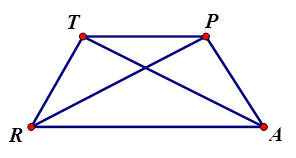# Warm-Up Trapezoids

Author:
powere
Topic:
Trapezoid

1. What are the properties of trapezoids? Check all that apply.

Check all that apply

2. What are the properties of isosceles trapezoids? Check all that apply.

Check all that apply3. TRAP is an isosceles trapezoid where m<RTP = 75 degrees, TR = 4, PR = 7. Fill in the blank: Segment TP is _____ to segment RA.

Check all that apply

﻿4. TRAP is an isosceles trapezoid where m<RTP = 75 degrees, TR = 4, PR = 7. Fill in the blank: Segment TR is _____ to segment PA, but not _____.

Check all that apply

﻿5. TRAP is an isosceles trapezoid where m<RTP = 75 degrees, TR = 4, PR = 7. m<TRA = _____

Check all that apply

﻿﻿6. TRAP is an isosceles trapezoid where m<RTP = 75 degrees, TR = 4, PR = 7. m<RAP = _____

Check all that apply

﻿﻿7. TRAP is an isosceles trapezoid where m<RTP = 75 degrees, TR = 4, PR = 7. m<APT = _____

Check all that apply

﻿﻿8. TRAP is an isosceles trapezoid where m<RTP = 75 degrees, TR = 4, PR = 7. m<PTR = _____

Check all that apply

﻿﻿9. TRAP is an isosceles trapezoid where m<RTP = 75 degrees, TR = 4, PR = 7. PA = _____

Check all that apply

﻿﻿10. TRAP is an isosceles trapezoid where m<RTP = 75 degrees, TR = 4, PR = 7. TA = _____

Check all that apply